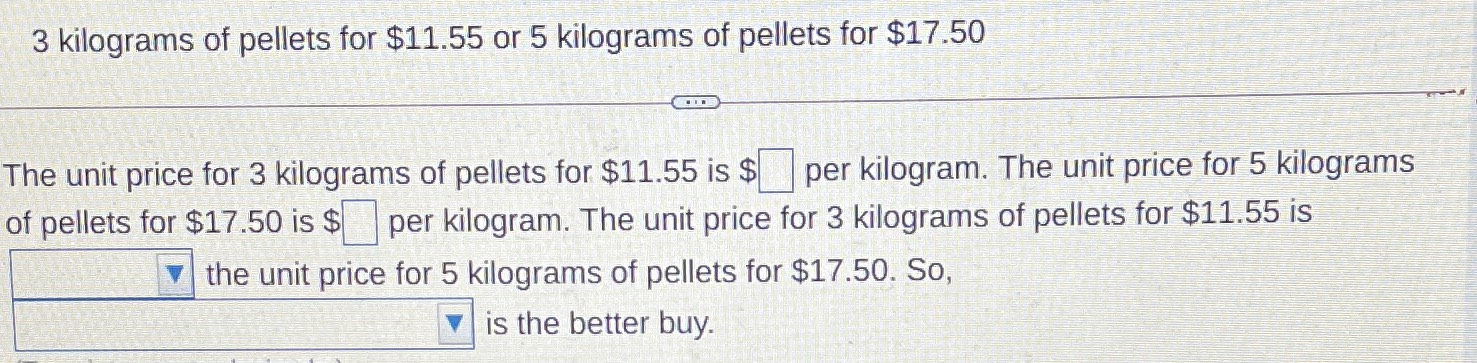### Still have math questions?

Arithmetic
Question$$3$$ kilograms of pellets for $$\ 11.55$$ or $$5$$ kilograms of pellets for $$\ 17.50$$

The unit price for $$3$$ kilograms of pellets for $$\ 11.55$$ is $$\ \square$$ per kilogram. The unit price for $$5$$ kilograms of pellets for $$\ 17.50$$ is $$\ \square$$ per kilogram. The unit price for $$3$$ kilograms of pellets for $$\ 11.55$$ is

$$\square$$ the unit price for $$5$$ kilograms of pellets for $$\ 17.50 .$$ So,

$$is the better buy.$$

The unit price for 3 kilograms of pellets for $11.55 is$3.85 per kg
The unit price for 5 kilograms of pellets for $17.50 is$3.5 per kg